# Some tiles associated with the 6th unit cubic Pisot number

## Windowed (Spiral, or Internal) Tile

There is a tile with the same outline as the symmetric tile, but with the smaller element embedded in one of the larger elements, leaving a spiral of voids ("windows") in the overall tile. Alternatively it can be viewed as a spiral of symmetric tiles. There are two alternative dissections. An IFS for the 1st dissection is { ```p → -ap - 1; p → -ap + 1; p → ap3 + 1 - a3``` } and for the 2nd is { ```p → -ap - 1; p → ap + 1; p → -ap3 + 1 + a3``` }.#### Tiling

As for the symmetric tile it is useful to introduce the numbers ```tn = an(3 - a - a2) = 4an/(1 - a3)``` to describe tilings.

It is intuitively obvious that it is not possible to tile the plane with only one copy of the tile in the unit cell. Three tilings with two copies in the unit cell, with signatures 00, 01 and 02, have been identified.

For the IFSs given above, the cell transforms for the unit cell of the 00 tiling are { ```p → p; p → -p + 1 - (t0 - t2) / 2``` } and the tiling vectors are ```t0 - t2``` and `t1 + t2` (or `t0 - t2` and ```t1 + t2```).

[For the alternative IFS { ```p → -ap; p → -ap + 1; p → ap3 + 1``` } the cell transforms take the simpler form { ```p → p; p → -p``` } and the tiling vectors are reduced in length by a factor of 2.]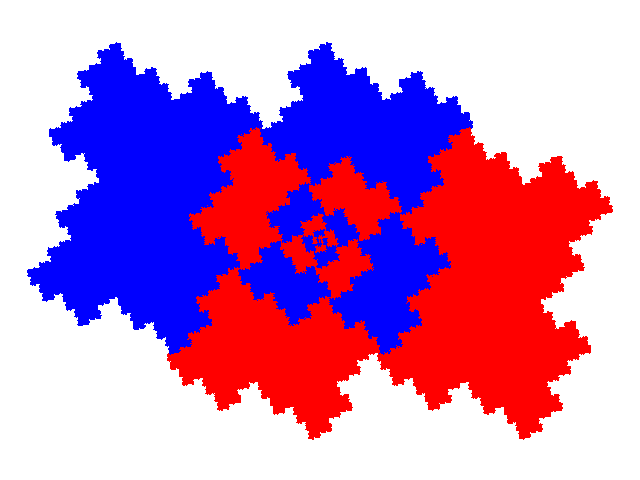The cell transforms for the unit cell of the 01 tiling are { ```p → p; p → ap + 1 + t2``` } and the tiling vectors are `t0` and `t1 + t2`.

[For the alternative IFS the cell transforms take the simpler form { ```p → p; p → ap``` } and the tiling vectors are again reduced in length by a factor of 2.]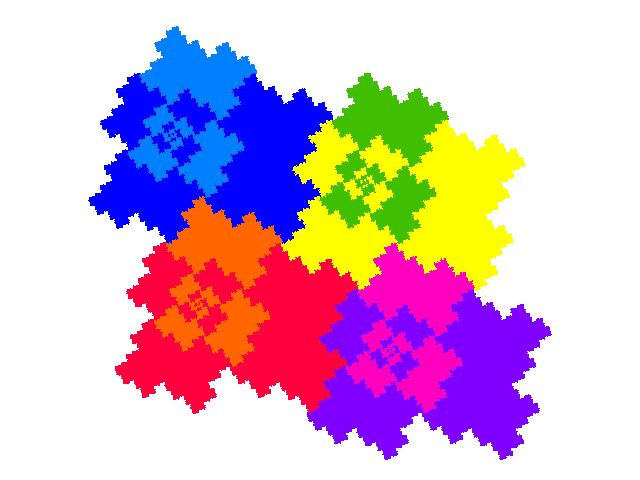The cell transforms for the unit cell of the 02 tiling are { ```p → p; p → -a2p + a2``` } and the tiling vectors are `t0` and `t1`.

[For the alternative IFS the cell transforms take the simpler form { ```p → p; p → -ap2``` } and the tiling vectors are again reduced in length by a factor of 2.]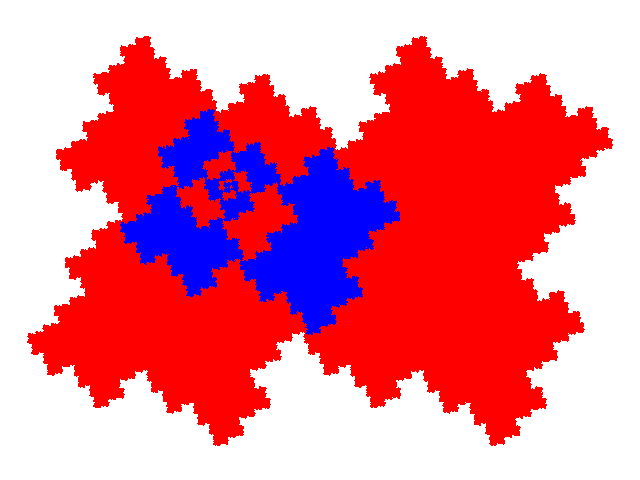Additional tilings can be produced by dissecting one or both elements of the unit cell. Thus from 00 we have tilings with signatures 0113 and 000022; from 01 tilings with signatures 0002, 0224 and 002113; and from 02 tilings with signatures 0012, 0335 and 002224. Obviously further dissections can be performed.

The unit cell for the 00 tiling is the same as one of the unit cells for the 012 tiling of the symmetric tile, the unit cell for the 01 tiling is the same as the unit cell for the 02 tiling of the symmetric tile, and the unit cell for the 02 tiling is the same as the unit cell for the 0 tiling of the symmetric tile. Consequently any tiling of the windowed tile can be converted into a tiling of the symmetric tile, and vice versa.

As there are two different dissections of the tile each dissection step leads to two different tilings. For example the two unit cells with signature 0335 are as shown below.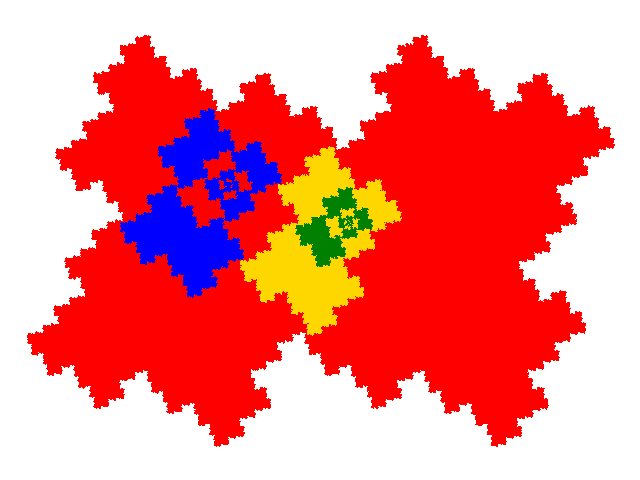Note that the 02 unit cell can replace a symmetric tile in two different orientations, with different selections of orientation give different tilings. Thus for the symmetric tile 0 tiling there are not only 02 tilings, but also three different 0202 tilings, with the orientation alternating along rows, along columns, or diagonally. Combining choice of dissection and orientation gives 8 0123 tilings based on the symmetric tile 01 tiling.

Pulling apart rows and/or columns and filling in the gaps is an alternative to convering tilings of the symmetric cell. Some sample "pull-apart" tilings (0002, 0011, 001111, 0012 and 2 versions of 001212) are shown below.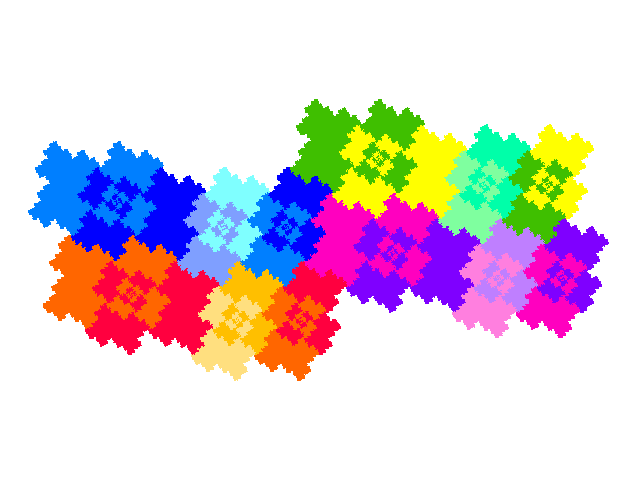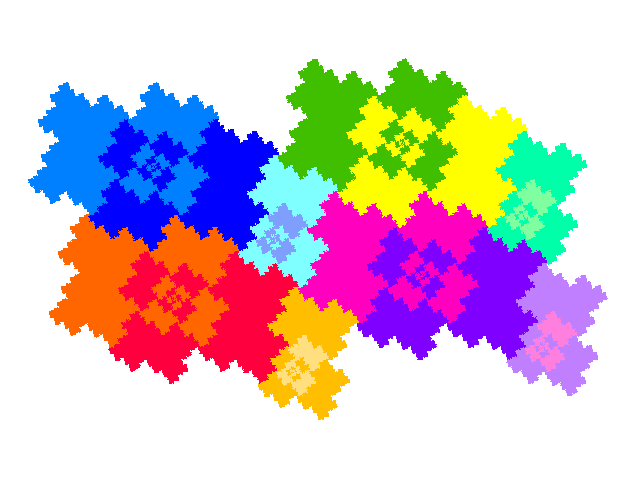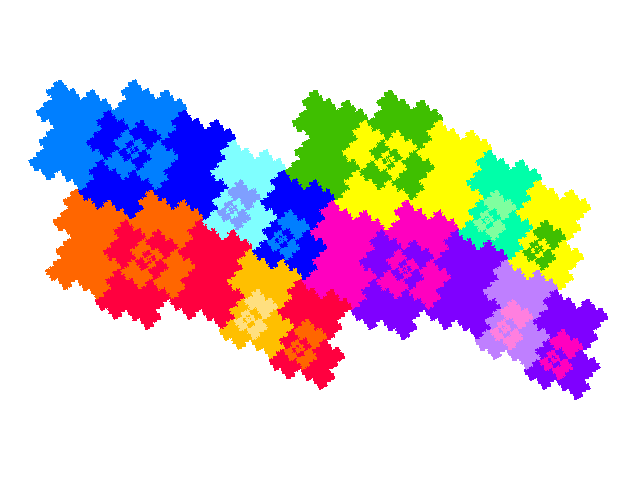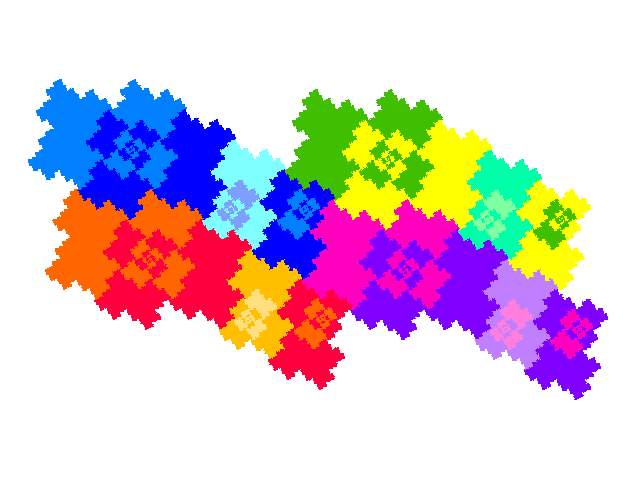© 2015, 2016 Stewart R. Hinsley